Sound - NCERT Questions
Q 1.

How does the sound produced by a vibrating object in a medium reach your ear?

SOLUTION:

When a disturbance is created on an object, it starts vibrating and sets the particles of the medium to vibrate. The particle of the medium is displaced from its mean position and exerts a force on the adjacent particle. As a result, the adjacent particle is disturbed from its mean position and the original particle comes back to rest. This process continues till the disturbance reaches our ear.

Q 2.

Explain how sound is produced by your school bell.

SOLUTION:

When the school bell is struck with a hammer, it starts vibrating and as a result of these vibrations, sound waves are produced.

Q 3.

Why are sound waves called mechanical waves?

SOLUTION:

Waves which need a material medium for propagation are called mechanical waves. Since sound waves also need a material medium for propagation, these waves are called mechanical waves. Sound wave are called mechanical waves or elastic waves as these are produced in a deformable or elastic medium. Unlike electromagnetic waves, sound waves need a medium to sustain them. Mechanical waves are governed by Newton’s laws of motion.

Q 4.

Suppose you and your friend are on the moon. Will you be able to hear any sound produced by your friend?

SOLUTION:

No we will not be able to hear the sound because sound requires a medium for its propagation. On the moon there is no atmosphere, i.e., there is vacuum.

Q 5.

Which wave property determines (A) loudness, (B) pitch?

SOLUTION:

(A) Loudness is determined by the amplitude of the sound wave which in turn depends on the force with which the object is made to vibrate.
(B) Pitch of a sound is determined by its frequency. (Apart from this, the pitch of sound also depends upon the relative motion between the source of sound and the listener).

Q 6.

Guess which has a higher pitch : a guitar or a car horn?

SOLUTION:

A guitar has a higher pitch than a car horn, provided the guitar is properly tuned.

Q 7.

What are wavelength, frequency, time period and amplitude of a sound wave?

SOLUTION:

Wavelength : The distance between two consecutive compressions (C) or two consecutive rarefactions (R) is called the wavelength, (unit metre).
Frequency : The number of oscillations per unit time is called frequency, unit (Hz).
Time period : The time taken by two consecutive compressions or rarefactions to cross a fixed point is called the time period.
Amplitude : The magnitude of the maximum disturbance in the medium on either side of the mean value is called the amplitude of the wave.

Q 8.

How are the wavelength and frequency of a sound wave related to its speed?

SOLUTION:

The speed is defined as the distance travelled by a wave per unit time.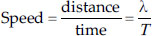λ is the wavelength, T → time period.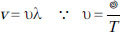∴ Speed = wavelength × frequency

Q 9.

Calculate the wavelength of a sound wave whose frequency is 220 Hz and speed is 440 m s–1 in a given medium.

SOLUTION:

Here, frequency of the sound wave, υ = 220 Hz speed of the sound wave, v = 440 m s–1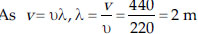Q 10.

A person is listening to a tone of 500 Hz sitting at a distance of 450 m from the source of the sound. What is the time interval between successive compressions from the source?

SOLUTION:

Here, frequency of the source, υ = 500 Hz time period of the tone,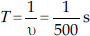The time period between successive compressions from the source is equal to the time period of the tone, i.e., (1/500) s and it has nothing to do with the distance (450 m) of the person from the source provided the sound wave reaches the person.

Q 11.

Distinguish between loudness and intensity of sound.

SOLUTION: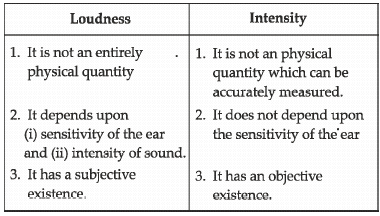Q 12.

In which of the three media, air, water or iron, does sound travel the fastest at a particular temperature?

SOLUTION:

The speed of sound in air = 346 m s–1.
The speed of sound in water = 1531 m s–1.
The speed of sound in iron = 5960 m s–1.
∴ The speed of sound in iron is greater and sound travels fastest in iron.

Q 13.

An echo returned in 3 s. What is the distance of the reflecting surface from the source, given that the speed of sound is 342 m s–1?

SOLUTION:

The speed of sound in air = 346 m s–1
Time taken by echo to return, t = 3 s
If d is the distance between the source and the reflecting surface, distance covered by sound in time t = d + d = 2d
(distance d while going to the reflecting surface and distance d while returning back)
As distance = speed (of sound) × time,
2d = 342 (m s–1) × 3 (s) = 1026 m
or d = 513 m

Q 14.

Why are the ceilings of concert halls curved?

SOLUTION:

The ceiling of the concert halls are curved to ensure that after reflection from the ceilings, sound reaches all corners of the hall.

Q 15.

What is the audible range of the average human ear?

SOLUTION:

20 Hz to 20,000 Hz.

Q 16.

What is the range of frequencies associated with
(A) Infrasound? (B) Ultrasound?

SOLUTION:

Range of frequencies associated with infrasound : 1 Hz to 20 Hz.
(B) Range of frequencies associated with ultrasound : 2 × 104 Hz to 1010 Hz.

Q 17.

A submarine emits a sonar pulse, which returns from an underwater cliff in 1.02 s. If the speed of sound in salt water is 1531 m s–1, how far away is the cliff?

SOLUTION:

Speed of sound in salt water = 1531 m s–1
Time period = 1.02 sec
Distance travelled 2 × d = speed × time
2 × d = 1531 × 1.02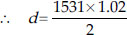d = 780 m

Q 18.

What is sound and how is it produced?

SOLUTION:

Sound is a form of energy and it is produced due to vibrations of different types of object, e.g., a vibrating tuning fork, a bell, wires in a sitar and a violin etc.

Q 19.

Describe with the help of a diagram, how compressions and rarefactions are produced in air near a source of sound.

SOLUTION:

(i) When a vibrating object moves forward, it pushes the air in front of it and compresses the air creating a region of high pressure called compression (C).
(ii) It starts moving away from the surface of the vibrating object.
(iii) As this occurs the surface moves backward creating a region of low pressure called rarefaction (R).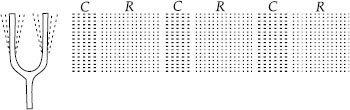Q 20.

Cite an experiment to show that sound needs a material medium for its propagation.

SOLUTION:

Take an electric bell and an air tight glass bell jar. The electric bell is suspended inside an air tight glass jar which is connected to a vacuum pump.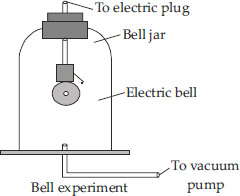Working : (i) When we press the switch, we will be able to hear the bell.
(ii) When the air in the jar is pumped out gradually, the sound becomes feeble although the same amount of current is flowing through the bell.
(iii) When the air is removed completely, we will not be able to hear the sound of the bell.
Conclusion : This experiment shows that sound requires a medium for its propagation.

Q 21.

Why is sound wave called a longitudinal wave?

SOLUTION:

A sound wave is called a longitudinal wave as it travels in a medium in the form of compressions and rarefactions where the particles of the medium vibrate in a direction which is parallel to the direction of propagation of the sound wave.

Q 22.

Which characteristic of the sound helps you to identify your friend by his voice while sitting with others in a dark room?

SOLUTION:

The quality (or timbre) of sound is that characteristic which enables us to distinguish one sound from the other even when these are of the same pitch and loudness. Each person has its own quality of sound and it is this characteristic which enables us to identify a person from others even without looking at him (i.e., in a dark room).

Q 23.

Flash and thunder are produced simultaneously. But thunder is heard a few seconds after the flash is seen, why?

SOLUTION:

The speed of light (c) is greater than the speed of sound (v) by a factor of 106 as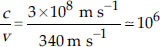Thus, the flash of light is seen earlier than the thunder of sound even though both are produced simultaneously.

Q 24.

A person has a hearing range from 20 Hz to 20 kHz. What are the typical wavelengths of sound waves in air corresponding to these two frequencies? Take the speed of sound in air as 344 m s–1.

SOLUTION:

Here, u1 = 20 Hz and u2 = 20 kHz = 20 × 103 Hz, speed of sound, v = 344 m s–1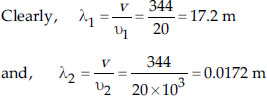Q 25.

Two children are at opposite ends of an aluminium rod. One strikes the end of the rod with a stone. Find the ratio of times taken by the sound wave in air and in aluminium to reach the second child.

SOLUTION:

Speed of sound in air = (v1) = 346 m s–1
Speed of sound in aluminium, v2 = 6420 m s–1
Let the length of the aluminium rod = x m
We know that speed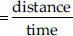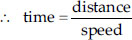Time taken in air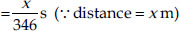Time taken in aluminium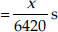Required ratio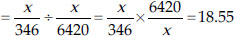Q 26.

The frequency of a source of sound is 100 Hz. How many times does it vibrate in a minute?

SOLUTION:

Frequency of sound = 100 Hz
Time taken = 1 minute = 60 s
We know frequency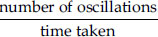∴ No. of oscillations = υ × t = 100 × 60 = 6000 times.

Q 27.

Does sound follow the same laws of reflection as light does? Explain.

SOLUTION:

Yes, sound follows the same laws of reflection as that of light because,
(i) Angle of incidence of sound is always equal to that of angle of reflection of sound waves.
(ii) The direction in which sound is incident, the direction in which it is reflected and normal all lie in the same plane.

Q 28.

When a sound is reflected from a distant object, an echo is produced. Let the distance between the reflecting surface and the source of sound production remains the same. Do you hear echo sound on a hotter day?

SOLUTION:

The minimum distance (d) for the distinct echo to be heard (say at 22°C) is 17.2 m (as 2d = vt = 344 × 0.1 = 34.4 m). On a hotter day, the temperature increases and the speed of sound in air also increases. For example, at 40°C, speed of sound, i.e. v 356 m s–1 and as such 2d = 356 × 0.1 = 35.6 m or d = 17.8 m.
Thus, if the distance of the reflecting surface and the source of sound remains the same (i.e., 17.2 m), no echo is heard on the hotter day as the minimum distance now required is 17.8 m.

Q 29.

Give two practical applications of reflection of sound waves.

SOLUTION:

Ear Trumpet : It is a sort of machine used by persons who are hard of hearing. The sound energy received by the wide end of the trumpet is connected into a much smaller area at the narrow end by multiple reflections. The narrow end of the trumpet which is inserted in the ear delivers the entire amount of energy falling on the wide end which makes the inaudible sound audible to the user.
Stethoscope : It is a medical instrument used frequently by doctors for making a rough diagnosis of the diseases existing inside the body at places which are either inaccessible or accessible only through major operations.

Q 30.

A stone is dropped from the top of a tower 500 m high into a pond of water at the base of the tower. When is the splash heard at the top? Given, g = 10 m s–2 and speed of sound is 340 m s–1.

SOLUTION:

Here, height through which the stone falls, h = 500 m
speed of sound, v = 340 m s–1, g = 10 m s–2
If t is the time taken by the stone to fall through h, then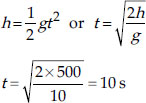Further, if t' is the time taken by sound (produced as a result of splashing) to travel to the top of the tower,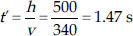Time after which the splash is heard at the top of the tower
= t + t' = 10 s + 1.47 s = 11.47 s

Q 31.

A sound wave travels at a speed of 339 m s–1. If its wavelength is 1.5 cm, what is the frequency of the wave? Will it be audible?

SOLUTION:

Here, speed of sound wave, v = 339 m s–1
Wavelength of sound wave,
λ = 1.5 cm = 1.5 × 10–2 m
Frequency of the sound wave,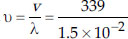= 22600 Hz
The sound is not audible as its frequency lies beyond the audible range
(20 Hz to 20000 Hz).

Q 32.

What is reverberation? How can it be reduced?

SOLUTION:

The persistence of sound in an auditorium as a result of repeated reflections of sound is called reverberation. To reduce the undesirable effects due to reverberation roofs and walls of the auditorium are generally covered with sound absorbent materials like compressed fiberboard, rough plaster, glass wool etc. The furniture is upholstered and floor is carpetted.

Q 33.

What is loudness of sound? What factors does it depend on?

SOLUTION:

Loudness of a sound depends on the amplitude of the vibration producing that sound. Greater is the amplitude of vibration, louder is the sound produced by it. The amplitude of the sound depends upon the force with which an object is made to vibrate.
The loudness of a sound also depends on the quantity of air that is made to vibrate.

Q 34.

Explain how bats use ultrasound to catch a prey.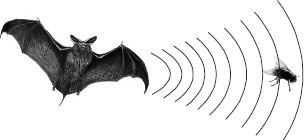SOLUTION:

The ultrasonic waves emitted by the bat are reflected from the prey (e.g., an insect) and are detected by its ears shown in figure. The nature of reflected waves tells the bat :
(i) the location and (ii) the nature of its prey.

Q 35.

How is ultrasound used for cleaning?

SOLUTION:

Ultrasound is used to clean parts located in hard-to-reach places (i.e.) spiral tube, odd shaped parks, electronic components etc. Objects to be cleaned are placed in a cleaning solutions and ultrasonic waves are sent into the solutions. Due to the high frequency, the dust particles, grease get detached and drop out. The objects thus get thoroughly cleaned.

Q 36.

Explain the working and application of a SONAR.

SOLUTION:

Working : SONAR consists of a transmitter and a detector and is installed in a boat or a ship as shown in figure.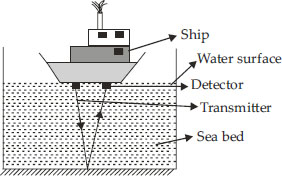The transmitter produces and transmits ultrasonic waves. These waves travel through water and after striking the object on the seabed, get reflected back and are sensed by the detector. The detector converts the ultrasonic waves into electrical signals which are appropriately interpreted. The distance of the object that reflected the sound wave can be calculated by knowing the speed of sound in water and the time interval between the transmission and reception of the ultrasound.
Let, depth of the sea = d
Speed of sound in sea water = v
Time taken for transmission and reception of signal = t
∴ Time taken to travel a distance,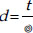∴ Depth of the sea,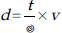( ... distance = speed × time)

Q 37.

A sonar device on a submarine sends out a signal and receives an echo 5 s later. Calculate the speed of sound in water if the distance of the object from the submarine is 3625 m.

SOLUTION:

Here, time interval between the transmission of the signal and its reception, t = 5 s
Distance of the object from the submarine, d = 3625 m
If v is the speed of sound in water, then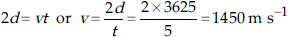Q 38.

Explain how defects in a metal block can be detected using ultrasound.

SOLUTION:

Ultrasounds can be used to detect cracks and flaws in metal blocks. Metallic components are used in the construction of big structures like buildings, bridges, machines and scientific equipments. The cracks or holes inside the metal blocks, which are invisible from outside reduces the strength of the structure. Ultrasonic waves are allowed to pass through the metallic block and detectors are used to detect the transmitted waves. If there is even a small defect, the ultrasound gets reflected back indicating the presence of the flaw or defect.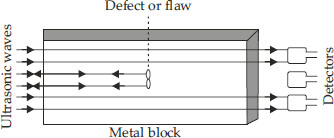Q 39.

Explain how the human ear works.

SOLUTION:

The function of the human ear is shown in figure.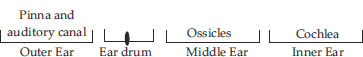(i) The outer ear collects sound wave which are conducted through the auditory canal.
(ii) These waves fall on the ear drum and set it into vibrations.
(iii) The middle ear which is of the size of a small marble and houses ossicles (three bones : hammer, anvil and stirrup) amplifies these oscillations about 60 times.
(iv) The inner ear which contains cochlea and is filled with a fluid converts these pressure variations into the electrical signals.
(v) These electrical signals are conveyed to the brain via auditory nerve for interpretation.